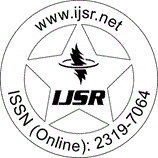International Journal of Science and Research (IJSR)
Call for Papers | Fully Refereed | Open Access | Double Blind Peer Reviewed

Research Paper | Mathematics | Iraq | Volume 5 Issue 11, November 2016

# Exact Solutions for the Mikhailov-Shabat Equation, and Classical Boussinesq Equation by Tan-Cot Method

Abstract: In this paper, we established a travelling wave solution by using the proposed Tan-Cot function algorithm for non-linear partial differential equations. The method is used to obtain new solitary wave solutions for non-linear partial differential equations such as, for the Mikhailov-Shabat ( MS) equation, and Classical Boussinesq (CB) equation, which are the important Soliton equations. Proposed method has been successfully implemented to establish new solitary wave solutions for the non-linear PDEs.

Keywords: Non-linear PDEs, Tan-Cot function method, Mikhailov-Shabat (MS) equation, Classical Boussinesq (CB) equation

Edition: Volume 5 Issue 11, November 2016,

Pages: 1971 - 1974